### Summary Merge-Time Data

Last GHCrawler update: 2020-08-02 09:48:20

Time until a typical PR has an 80% chance of being merged

### Per-Label Merge-Time Data

PRs open / merged in the last year

Survival analysis can be tricky to interpret. This document helps to makes sense of what you see in the rest of the app.

## What is Survival Analysis?

Survival Analysis refers to the study of any time-to-event data. Traditionally this was the study of patients in a hospital, which is where the name comes from, but here we are studying the time-to-merge of Pull Requests.

The key factor in survival analysis is that we have incomplete data. A PR that is merged is in a fully known state - but a PR that is open might be merged later. This is known as censored data, and can be seen a bit like this: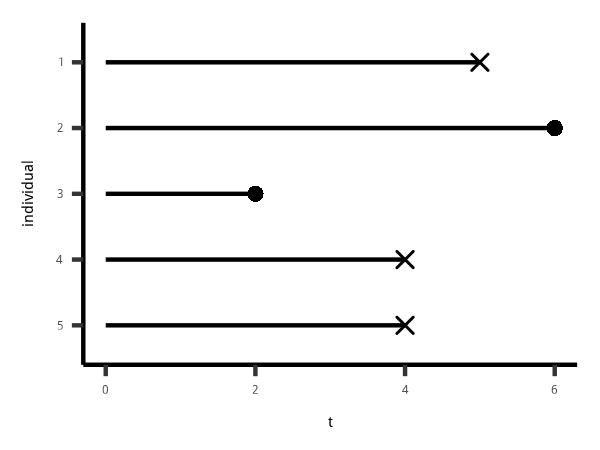The circles are known cases where the PR was merged (or the patient died, etc), and the crosses are where the PR is still open (patient still alive, etc) at the time of the study. It might be merged later, it might never be merged - we don't know.

For this reason, measures such as the median applied to the merge times of PRs will tend to underestimate the true value, as it doesn't account for the amount of time that currently open PRs remain open into the future.

If we think about visualising the same data in the form of “how many PRs are left open?” as a function of time, it would look like this: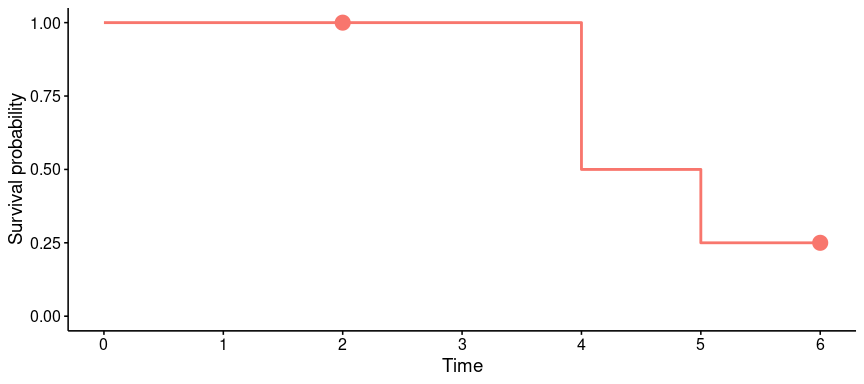Note how at time `t = 2` we lose a “patient” (a PR) from the study, but we still have 100% survival - that's because instead of having 5 out of 5 things to track, we've gone down to 4, but it's still 4 out of 4. This happens where a PR is still open, but quite new - since we're tracking “Time since opened”, a PR opened a week ago will censor out at ```t = 7```, as we can't know any more about it after that.

Let's see a more realistic survival curve…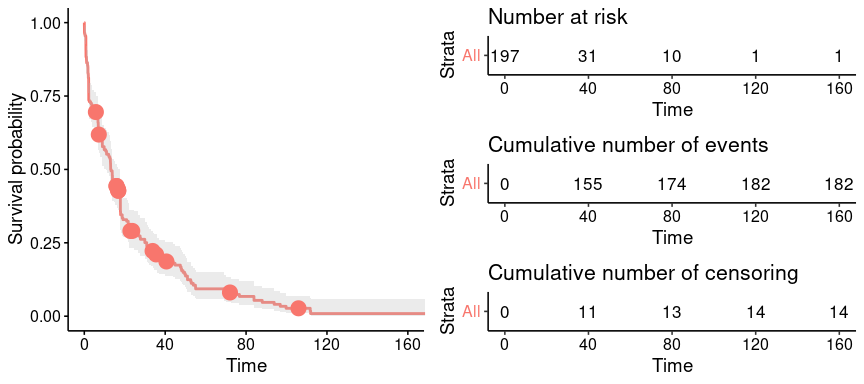This is for the `netapp` label in `ansible/ansible`, as of 2019-04-03. There are 197 PRs, and at `t = 0`, we (of course) have 100% of the PRs left in the set. As time advances, PRs either censor out, or are merged - as the tables below show, by 120 days, only 1 PR is left, with 182 having been merged, and 14 censored, i.e still open (the last one eventually censors out at 172 days).

## Making predictions

The above curve is quite “bumpy” as it's a step function (called the Kaplan-Meier estimate) - for predictions, we want a smooth function. For that, we use a model called the Weibull distribution. Here's the same data with the Weibull model on top: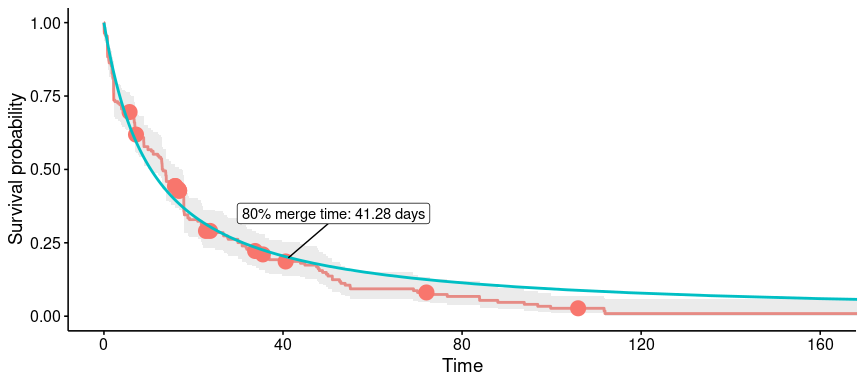We can see how the Weibull model gives a smooth predictive function, which we can use to ask questions like “What's the time needed to reach a 20% chance of survival?”. These are what you can see in the app.

## Why do I see unrealistic values like 2000 years?!

Recall that the Weibull model provides a smooth curve for a given dataset. Now ask yourself what happens if the dataset has a large percentage of censored (i.e. still open) PRs?

Here's an example from the `affects_2.4` label: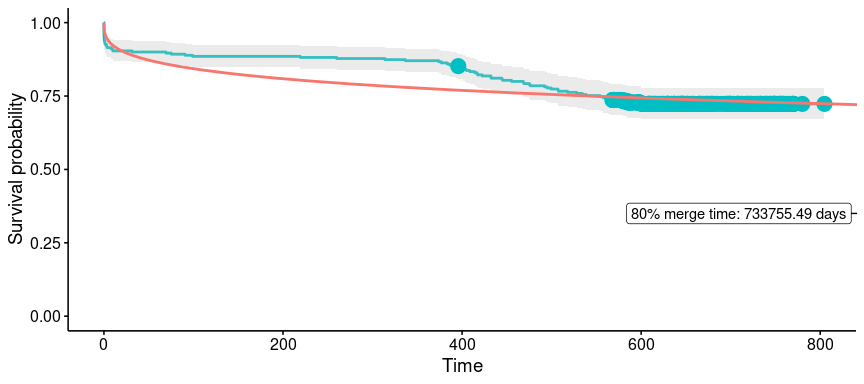See how the curve is very high up, and the slope is very slight? That means it takes a very long time to reach the 20% chance of survival point on the curve. Thus the interpretation of an absurdly high 80% merge time is that there are many open PRs in that label (on in other words, the problem is in the data, not the analysis!)